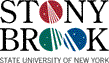###Department of Mathematics SUNY at Stony Brook

MAT 126 home | Syllabus | Schedule | Practice Exams | Exam Scores | Instructors

# Math 126 - Fall 2006

Here is the practice exam for the MAT 126 final exam in Spring 2006.
And here are the solutions for that practice exam.
Of course, this practice exam does not cover all possible topics that could be tested on our exam. For example, it does not cover section 6.5.

Here is the practice exam for the second MAT 126 midterm in Spring 2006.
And here are the solutions for that practice exam.
Note: it says on the practice exam that "The actual exam will contain 5 problems". That was true in Spring 2006 but may not be true about our own midterm.
Of course, this practice exam does not cover all possible topics that could be tested on our midterm. For example, it does not cover section 5.7.

Here is the practice exam for the first MAT 126 midterm in Spring 2006.
And here are the solutions for that practice exam.
Of course, these practice exams do not cover all possible topics that could be tested on our midterm. For instance, this practice exam did not have any questions testing you on the various meanings of the integral (as in the problems on page 375 of the book).